ggrastr

Rasterizing ggplot objects with rasterize

When you need to plot data with many observations, storing plots completely in vector format is unsuitable: it requires tons of space and is very slow to work with in graphic editors. On the other hand, completely rasterizing the plot distorts important text content and prevents readers from editing and copying. The solution provided here within the package ggrastr is to rasterize layers that have a lot of data points, keeping all the rest in vector format.

The simplest way to rasterize some of your layers is to call rasterize on the plot object:

library(ggplot2)
library(ggrastr)

plot <- ggplot(diamonds, aes(carat, price, colour = cut)) +
geom_point()

rasterize(plot, layers='Point', dpi=50)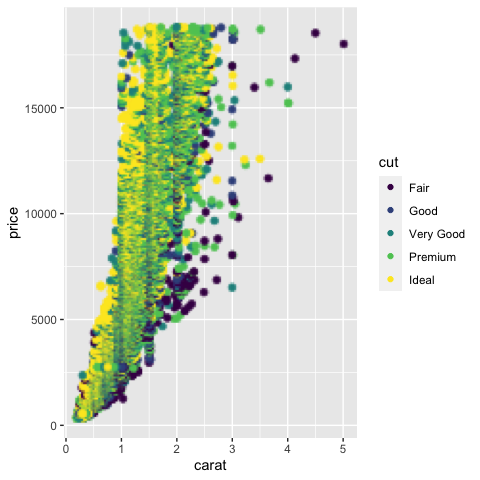The layer parameter here accepts the layers, which should be rasterized and can work with vectors of layer types.

Rasterizing individual layers

The same function can be applied on the level of individual layers. It allows users to rasterize only some layers of the same type:

ggplot() +
rasterise(geom_point(aes(carat, price, colour = cut), data=diamonds), dpi=30) +
geom_point(aes(x=runif(20, 0, 5), y=runif(20, 0, 20000)), size=10, color="black", shape=8)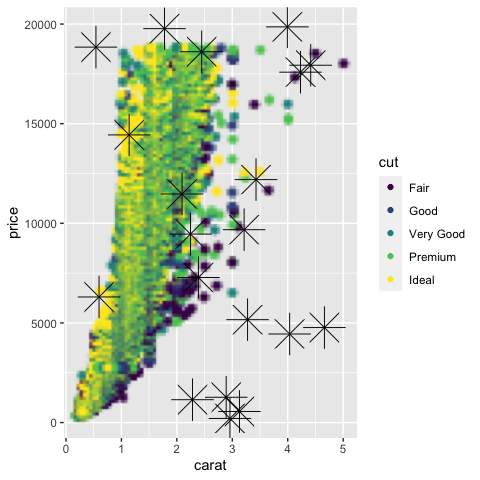Note that when the aspect ratio is distorted, the objects are rendered without distortion, i.e. the points in this example are still circles:

# Points remain round across different aspect ratios
plot <- ggplot(diamonds, aes(carat, price, colour = cut))
plot + rasterise(geom_point(), dpi = 72) + theme(aspect.ratio = 0.2)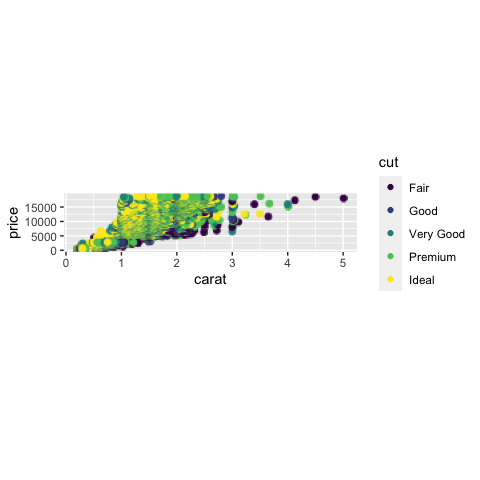Selecting rendering device

By default, plots are rendered with Cairo. However, users now have the option to render plots with the ragg device. The motivation for using ragg is that ragg can be faster and has better anti-aliasing. That being said, the default ragg device also has some alpha blending quirks. Because of these quirks, users are recommended to use the ragg_png option to work around the alpha blending.

The differences in devices are best seen at lower resolution:

# The default 'cairo' at dpi=5
plot + rasterise(geom_point(), dpi = 5, dev = "cairo")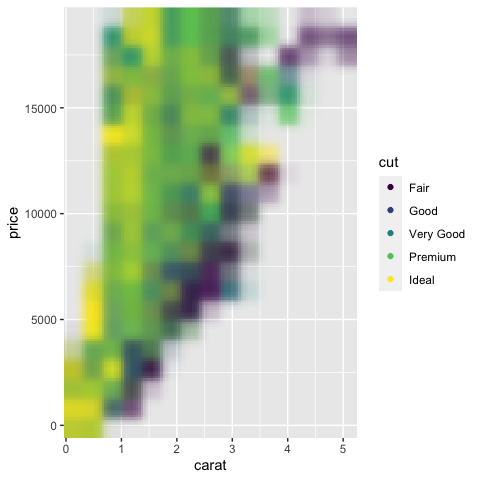# Using 'ragg' gives better anti-aliasing but has unexpected alpha blending
plot + rasterise(geom_point(), dpi = 5, dev = "ragg")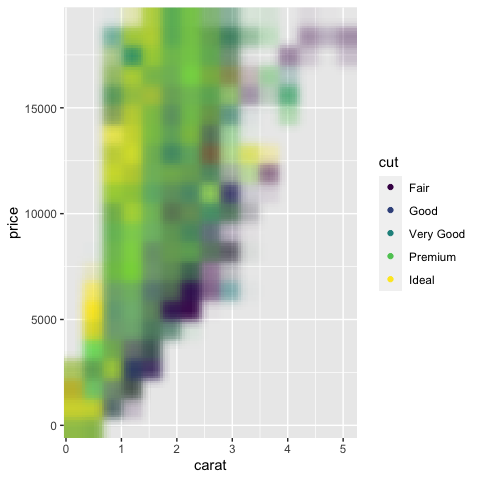# Using 'ragg_png' solves the alpha blend, but requires writing a temporary file to disk
plot + rasterise(geom_point(), dpi = 5, dev = "ragg_png")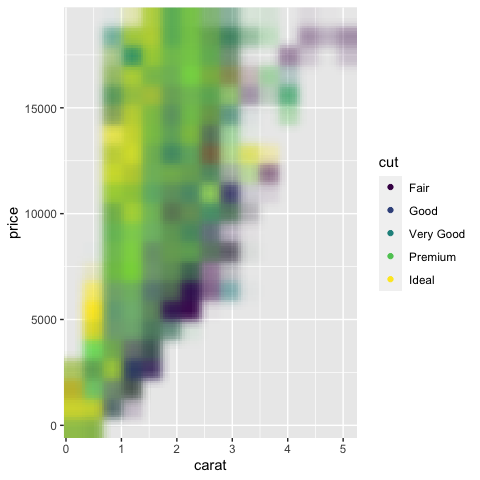Note that facets are rendered correctly without users having to adjust the width/height settings.

# Facets will not warp/distort points
set.seed(123)
plot + rasterise(geom_point(), dpi = 300) + facet_wrap(~ sample(1:3, nrow(diamonds), 2))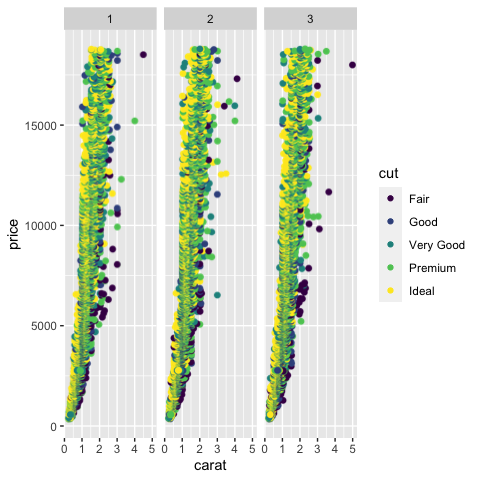Scaling size of raster objects

Users are also able to change the size of the raster objects with the parameter scale. The default behavior is not to modify the size with scale=1:

# unchanged scaling, scale=1
plot <- ggplot(diamonds, aes(carat, price, colour = cut))
plot + rasterise(geom_point(), dpi = 300, scale = 1)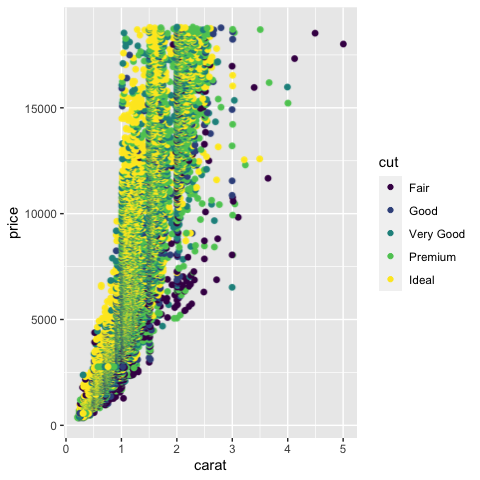Setting scale to values greater than 1 will increase the size of the rasterized objects. In this case, scale=2 will double the size of the points in comparison to the original plot:

# larger objects, scale > 1
plot <- ggplot(diamonds, aes(carat, price, colour = cut))
plot + rasterise(geom_point(), dpi = 300, scale = 2)